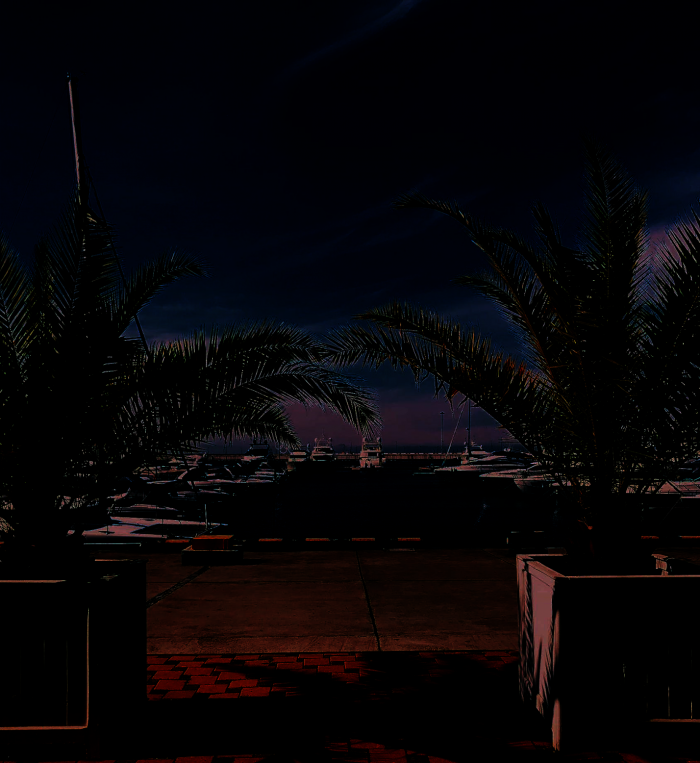# How to apply a gamma correction to a Graphics Draw (GD) image in PHP?

imagegammacorrect() is an inbuilt function in PHP that is used to apply a gamma correction to a given Graphics Draw (GD) input image and an output gamma.

bool imagegammacorrect(resource $image, float$inputgamma, float $outputgamma) ## Parameters imagegammacorrect() takes three different parameters:$image, $inputgamma and$outputgamma.

• $image − Specifies the image to be worked. •$inputgamma − Specifies the input gamma.

• $outputgamma − Specifies the output gamma. ## Return Values imagegammacorrect() returns True on success and False on failure. ## Example 1 <?php // load an image from the local drive folder$img = imagecreatefrompng('C:\xampp\htdocs\Images\img58.png');

// Change the image gamma by using imagegammacorrect
imagegammacorrect($img, 15, 1.5); // Output image to the browser header('Content-Type: image/png'); imagepng($img);
imagedestroy(\$img);
?>

## Output

Input image before using imagegammacorrect() PHP functionOutput image after using imagegammacorrect() PHP functionExplanation − In this example, we loaded the image from the local drive folder by using the imagecreatefrompng() function or we can also use the URL of an image. After that, we applied imagegammacorrect() with the values 5 and 1.5. We can see the difference between the two images in the output.